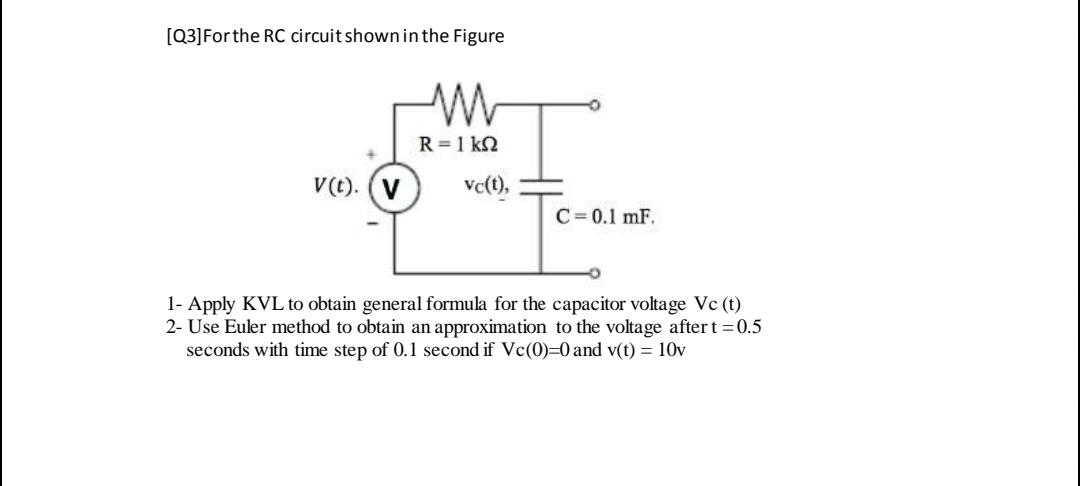# [Q3]Forthe RC circuit shown in the Figure R =1 kN V(t). (V ve(), C= 0.1 mF. 1- Apply KVL to obtain general formula for the capacitor voltage Vc (t) 2- Use Euler method to obtain an approximation to the voltage after t = 0.5 seconds with time step of 0.1 second if Vc(0)=0 and v(t) = 10v

Questionhelp_outlineImage Transcriptionclose[Q3]Forthe RC circuit shown in the Figure R =1 kN V(t). (V ve(), C= 0.1 mF. 1- Apply KVL to obtain general formula for the capacitor voltage Vc (t) 2- Use Euler method to obtain an approximation to the voltage after t = 0.5 seconds with time step of 0.1 second if Vc(0)=0 and v(t) = 10v fullscreen

### Want to see this answer and more?

Experts are waiting 24/7 to provide step-by-step solutions in as fast as 30 minutes!*

*Response times may vary by subject and question complexity. Median response time is 34 minutes for paid subscribers and may be longer for promotional offers.
Tagged in
Engineering
Electrical Engineering

### Circuit Theory